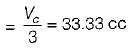Courses

# Basics Of IC Engine - 2

## 10 Questions MCQ Test Mock Test Series - Mechanical Engineering (ME) for GATE 2020 | Basics Of IC Engine - 2

Description
This mock test of Basics Of IC Engine - 2 for Mechanical Engineering helps you for every Mechanical Engineering entrance exam. This contains 10 Multiple Choice Questions for Mechanical Engineering Basics Of IC Engine - 2 (mcq) to study with solutions a complete question bank. The solved questions answers in this Basics Of IC Engine - 2 quiz give you a good mix of easy questions and tough questions. Mechanical Engineering students definitely take this Basics Of IC Engine - 2 exercise for a better result in the exam. You can find other Basics Of IC Engine - 2 extra questions, long questions & short questions for Mechanical Engineering on EduRev as well by searching above.
QUESTION: 1

### Consider the following statements: 1. Mean piston speed is given by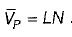2. Automobile engines operate at higher end of mean piston speed near to 15 m/s. 3. Large marine diesel operate at lower end of mean piston speed near to 8 m/s. Which of these are correct?

Solution:

Mean piston speed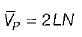QUESTION: 2

### All of followings are correct except: Inlet-valve mach index is dependent on

Solution: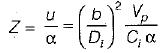where

u = gas velocity through the inlet valve at smallest flow area
α = inlet sonic velocity
b = bore
Di = inlet valve diameter
Vp = mean piston speed
Ci = inlet valve average flow coefficient

QUESTION: 3

### The mechanical efficiency of a single-cylinder four-stroke engine is 80%. The frictional power is estimated to be 20 kW. What is the brake power developed by the engine?

Solution: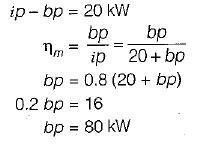QUESTION: 4

Piston compression rings are made of

Solution:
QUESTION: 5

The minimum number of rings in a piston are

Solution:

The leakage of gases between the walls of piston and cylinder is prevented by means of three to six cast iron rings which may be square or rectangular in cross-section.

QUESTION: 6

The slotted grooves on oil rings for pistons help to

Solution:
QUESTION: 7

Match the List-I (IC engine parts) with List-il (Materials) following: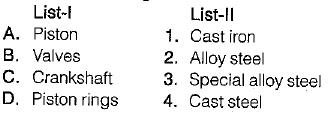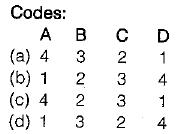Solution:
QUESTION: 8

The top of the piston in two-stroke engine is

Solution:

It is for scavenging of air.

QUESTION: 9

Accumulation of carbon deposits on the cylinder head of an IC engine leads to increase in

Solution:
QUESTION: 10

The engine of a car has three cylinder with total displacement of 770 cc. The compression ratio is 8.7. What is the clearnace volume of each cylinder?

Solution: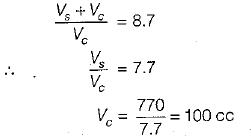Clearance volume of each cylinder